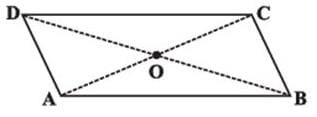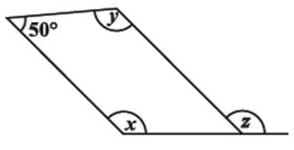Test Description

## 10 Questions MCQ Test Mathematics (Maths) Class 8 | Test: Understanding Quadrilaterals- 1

Test: Understanding Quadrilaterals- 1 for Class 8 2022 is part of Mathematics (Maths) Class 8 preparation. The Test: Understanding Quadrilaterals- 1 questions and answers have been prepared according to the Class 8 exam syllabus.The Test: Understanding Quadrilaterals- 1 MCQs are made for Class 8 2022 Exam. Find important definitions, questions, notes, meanings, examples, exercises, MCQs and online tests for Test: Understanding Quadrilaterals- 1 below.
Solutions of Test: Understanding Quadrilaterals- 1 questions in English are available as part of our Mathematics (Maths) Class 8 for Class 8 & Test: Understanding Quadrilaterals- 1 solutions in Hindi for Mathematics (Maths) Class 8 course. Download more important topics, notes, lectures and mock test series for Class 8 Exam by signing up for free. Attempt Test: Understanding Quadrilaterals- 1 | 10 questions in 10 minutes | Mock test for Class 8 preparation | Free important questions MCQ to study Mathematics (Maths) Class 8 for Class 8 Exam | Download free PDF with solutions
 1 Crore+ students have signed up on EduRev. Have you?
Test: Understanding Quadrilaterals- 1 - Question 1

### Which of the following quadrilaterals has two pairs of adjacent sides equal and diagonals intersecting at right angles?

Detailed Solution for Test: Understanding Quadrilaterals- 1 - Question 1

Explanation:- A rhombus is a  parallelogram and a quadrilateral whose opposite sides are parallel and opposite angles are equal.

Area of Rhombus, A = (d1 x d2)/2

The perimeter of Rhombus, P = 4a units

The opposite angles of a rhombus are equal to each other.

In a rhombus, diagonals bisecting each other at right angles.

Diagonals bisect the angles of a rhombus

Test: Understanding Quadrilaterals- 1 - Question 2

### If the sides of a triangle are produced in order,What is the sum of the exterior angles so formed?

Detailed Solution for Test: Understanding Quadrilaterals- 1 - Question 2

Suppose you have a ∆ABC
All the exterior angles will be equal to
(180-A)°
(180-B)°
(180-C)°
respectively (Angles on the same line) so if you sum it up it will be equal to 540 - (A+ B + C )
And by angle sum property A+B+C = 180°
Therefore Sum = 540 - 180 = 360°

Test: Understanding Quadrilaterals- 1 - Question 3

### Given a parallelogram ABCD. AD = ....Detailed Solution for Test: Understanding Quadrilaterals- 1 - Question 3 BC ( because of opposite sides of the parallelogram are equal = )
Test: Understanding Quadrilaterals- 1 - Question 4

The sum of the measures of the three angles of a triangle is ______.

Detailed Solution for Test: Understanding Quadrilaterals- 1 - Question 4

Angle sum property of a triangle is that sum of 3 angles is 180º

Test: Understanding Quadrilaterals- 1 - Question 5

The ________ of a rhombus are perpendicular bisectors of one another.

Test: Understanding Quadrilaterals- 1 - Question 6

Find the value of the unknown x in this parallelogram.Test: Understanding Quadrilaterals- 1 - Question 7

The opposite sides of a parallelogram are of ________ length.

Detailed Solution for Test: Understanding Quadrilaterals- 1 - Question 7

It’s a property of parallelogram that opposite sides of a parallelogram are equal and parallel.

Test: Understanding Quadrilaterals- 1 - Question 8

Which of the parallelograms has all sides equal and diagonals bisect each other at right angle?

Detailed Solution for Test: Understanding Quadrilaterals- 1 - Question 8

If two adjacent sides of a parallelogram are equal, then it is a rhombus.. A quadrilateral whose diagonals bisect each other at right angles is a rhombus.

In square all sides are equal and every angle is right angle.

Test: Understanding Quadrilaterals- 1 - Question 9

Which of the following quadrilaterals has two pairs of adjacent sides equal and diagonals intersecting at right angles?

Detailed Solution for Test: Understanding Quadrilaterals- 1 - Question 9

Rhombus, is a quadrilaterals has two pairs of adjacent sides equal and diagonals intersecting at right angles. A rhombus is a a parallelogram and a quadrilateral whose opposite sides are parallel and opposite angles are equal.

Test: Understanding Quadrilaterals- 1 - Question 10

State the name of a regular polygon of 5 sides.

Detailed Solution for Test: Understanding Quadrilaterals- 1 - Question 10

Pentagon have 5 sides .

## Mathematics (Maths) Class 8

187 videos|321 docs|48 tests
 Use Code STAYHOME200 and get INR 200 additional OFF Use Coupon Code